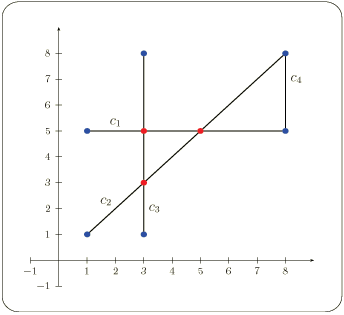# Chapter 252D Sweep Line of Planar Curves

Ester Ezra and Tali Zvi

## 25.1   Introduction

Let C:={c1,c2, ...cn} be a set of curves for which we want to compute all intersections. We want to avoid testing pairs of curves that are far apart. To find the intersecting pairs we imagine sweeping a line l from left to right over the plane, starting from a position left to all curves. While we sweep the plane, we keep track of all curves intersecting it.

This type of algorithm is called a plane sweep algorithm and the line l is called the sweep line. The status of the sweep line is the set of curves intersecting it. The status changes while the sweep line moves to the right, but not continuously. The sweep line status is updated at specific points called the event points. These points are actually the endpoints of all curves and their intersection points. The initial set of event points are only the endpoints of the curves. More event points are added as intersection points are calculated.

This chapter describes the Sweep_line_2 class it implements the plane sweep algorithm, and can be used to compute the intersection points of a given collection curves, the disjoint-interior subcurves induced by such a collection, and a few other retaled tasks.

In particular, we take advantage of the implemented sweep line algorithm to construct a Planar Map of intersecting curves efficiently. See CGAL::Pm_with_intersection for more deatils.

### 25.1.1   Functionality

Given a collection C of possibly intersecting (not necessarily x-monotone 1) curves in a plane, the Sweep_line_2 class provides the following operations that can be performed on C:

• calculate the intersection points between the curves of C.
• calculate the interior-disjoint curves induced by C.
• calculate the intersection points and return a list of curves participating at each intersection point.
• answer the query whether an intersection point between any two curves exists.

### 25.1.2   A Simple Program

The simple program listed below computes all sub segments induced by a set of four input segments illustrated in figure. The endpoints of the resulting sub segments are printed out.

Figure:  Four input segments```#include <CGAL/Cartesian.h>
#include <CGAL/MP_Float.h>
#include <CGAL/Quotient.h>
#include <CGAL/Arr_segment_cached_traits_2.h>
#include <CGAL/Sweep_line_2.h>
#include <vector>

typedef CGAL::Quotient<CGAL::MP_Float>                  NT;
typedef CGAL::Cartesian<NT>                             Kernel;
typedef CGAL::Arr_segment_cached_traits_2<Kernel>       Traits;
typedef Traits::Point_2                                 Point_2;
typedef Traits::Curve_2                                 Curve_2;
typedef std::list<Curve_2>                              CurveList;
typedef CurveList::iterator                             CurveListIter;
typedef CGAL::Sweep_line_2<CurveListIter, Traits>       Sweep_line;

int main()
{
CurveList  segments;
Curve_2 c1(Point_2(1,5), Point_2(8,5));
Curve_2 c2(Point_2(1,1), Point_2(8,8));
Curve_2 c3(Point_2(3,1), Point_2(3,8));
Curve_2 c4(Point_2(8,5), Point_2(8,8));

segments.push_back(c1);
segments.push_back(c2);
segments.push_back(c3);
segments.push_back(c4);

std::list<Curve_2> subcurves;
Sweep_line sl;
sl.get_subcurves(segments.begin(), segments.end(),
std::back_inserter(subcurves), true);

for (std::list<Curve_2>::iterator scv_iter = subcurves.begin();
scv_iter != subcurves.end(); scv_iter++)
std::cout << *scv_iter << std::endl;
return 0;
}
```

The input segments intersect at three interior points and meet at two endpoints. The program results with ten sub segments. The output of the program follows:

```1/1 1/1 -147/-49 -147/-49
1/1 5/1 -147/-49 -245/-49
3/1 1/1 -147/-49 -147/-49
-147/-49 -147/-49 -147/-49 -245/-49
-147/-49 -245/-49 3/1 8/1
-147/-49 -147/-49 245/49 245/49
-147/-49 -245/-49 245/49 245/49
245/49 245/49 8/1 5/1
245/49 245/49 8/1 8/1
8/1 5/1 8/1 8/1
```

## 25.2   Software Design

The Sweep_line_2<InputIterator,Traits> class is parameterized with two objects. The sequence of input curves are obtained through the InputIterator. The type of curves processed by the Sweep_line_2<InputIterator,Traits> class is dictated by the injected traits class, a model of the SweepLineTraits concept. Inject the suitable traits class, to handle your desired family of curves. The planar map with intersection comes with a collection of traits classes that handle various types of curves, such as segments, polylines, and conics. Naturally, users may author new traits classes that handle other types or that possesses different carachteristics.

### 25.2.1   Traits Classes

The Sweep_line_2<InputIterator,Traits> class is parameterized by a traits class, that defines the abstract interface between the sweep-line algorithm and the primitive it uses. It must define three types of objects, namely Curve_2, X_monotone_curve_2, and Point_2, where the type of the endpoints of an X_monotone_curve_2-type curve is Point_2. In addition, the traits class must provide a set of operations on these two types.

The PlanarMapWithIntersectionsTraits_2 concept is a refinement of the SweepLineTraits_2 concept, as some of the functions required to perform some of the planar map operations are not needed, by the sweep line algorithm. Hence, all the models of the Planar Map With Intersections Traits PlanarMapWithIntersectionsTraits_2 concept are also models of the SweepLineTraits_2 concept.

## 25.3   Implementation

The sweep line algorithm was implemented according to Bentley and Ottmann [dBvKOS97]. The implementation is robust. It supports general curves and handles all degenerate cases, including overlapping curves, vertical segments, and tangency between curves.

The STL map-container is used for the implementation of the event queue, and the STL set-container is used for the implementation of the status line. Additional containers are used to accomodate all intersection points for each input curve ordered from left to right, and all the resulting subcurves for each event point.

The complexity of this algorithm is O(nlogn + klogn) where n is the number of the input curves and k is the number of intersection points induced by these curves.

### Footnotes

 1 We stress this fact, because some implementation, such as, Planar_map_2 assume all curves are x-monotone in order to gain simplicity and speed.